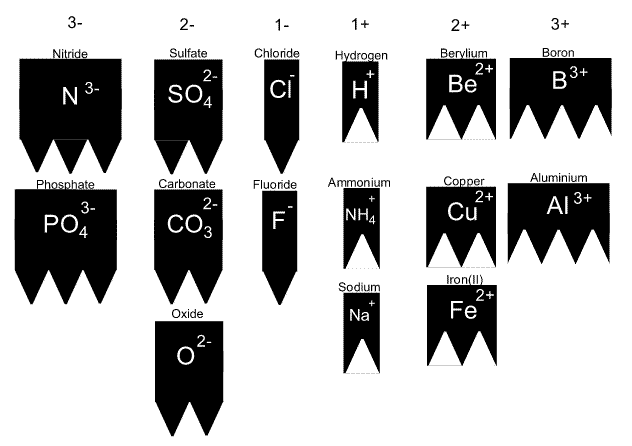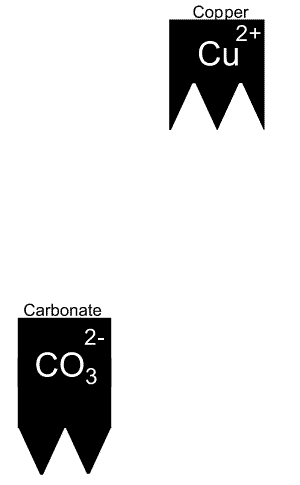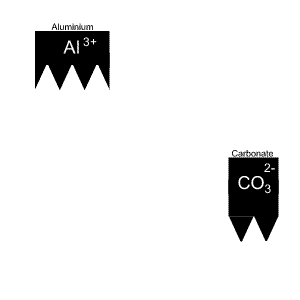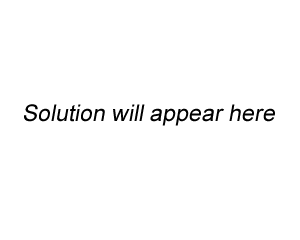Ionic formulae exercise By making cards of each ion listed above as shown, it is easy to visualise the compounds formed from two given ions. For example Give the name and formula of the compound formed when copper and carbonate react. The solution is animated on the right. We combine the ions until they are perfectly matched. In this case one of each ion is all it takes. The formula is therefore given as CUCO3. To name the compound we always put the positive ion first.Give the name and formula of the compound formed when aluminium and carbonate react. The solution is animated on the right. It takes two aluminium and three carbonate ions to complete the compound. Now, if we need more than one ion we need to use subscripts. We use brackets only if both of the conditions below exist: - the ion has more than one capital letter and - more than one ion is needed. Aluminium has only one capital letter so we do not need brackets. But carbonate has two capital letters so we need brackets.Give the names and formulae of the compounds formed when the following ions react. Chloride(Cl- ) and aluminium (Al3+) SolutionAmmonim (NH4+) and chloride (Cl- ) Carbonate (SO42-) and aluminium (Al3+) Solution Copper (Cu2+ ) and phosphorus (P3-) Why do we not get a reaction between two negative or two positive ions? The formula for ammonium phosphate, a very important fertiliser, is (NH4)3PO4 . Why is the ammonium ion in brackets and the phosphate is not?